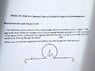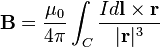# Biot-Savart law and Ampere's law

• slayer1337
In summary, the conversation discusses the determination of the magnetic field at a point P in the centre of a loop with a radius r, connected by two long straight sections with a gap subtending an angle of 60 degrees. The Biot-Savart law is used to calculate the magnetic field, and the equation for integrating dl/r^2 is discussed. The final solution involves using Ampere's law and the Biot-Savart law to calculate the field due to a continuous wire and a short section of wire connecting the loop.

#### slayer1337

1. An electric circuit consists of two long straight sections connecting a loop of radius r. The gap in the loop where the straight sections are connected subtends an angle of theta=60d, as shown in this figure (http://imgur.com/tseDHL6). Determine the magnetic field B at the point P in the centre of the loop, if a current I is flowing through the circuit.

See the diagram here - http://imgur.com/tseDHL6

2. Biot-Savart Law

3. The Attempt at a Solution - http://imgur.com/QFrlSn7

I have found the equation relating to the full loop but don't know how to do it for the missing segment.

#### Attachments

•photo.jpg
43 KB · Views: 473
What's wrong with integrating ##d l\over r^2## ?

You need to use the Biot-Savart law:Ma is right, but the next step is to convert to integrating ##d l\over r^2## because there is only one component left over; the others cancel.

$\int rd θ/r^2/$

Consider finding the field as though the circular section wasn't there, but the wire was continuous from -infinity to +infinity: B1. Can use ampere's law.

Then, use Biot-Savart to compute the field due to a short section of wire connecting the loose ends of the loop: B2.

Then, use Biot-Savart to compute the field due to the loop: B3.

B = B1 - B2 + B3.